Custom

# How to Make a Servo DemultiplexerJuly 05, 2021 by GreenPAK™ Family of Integrated Circuits
Share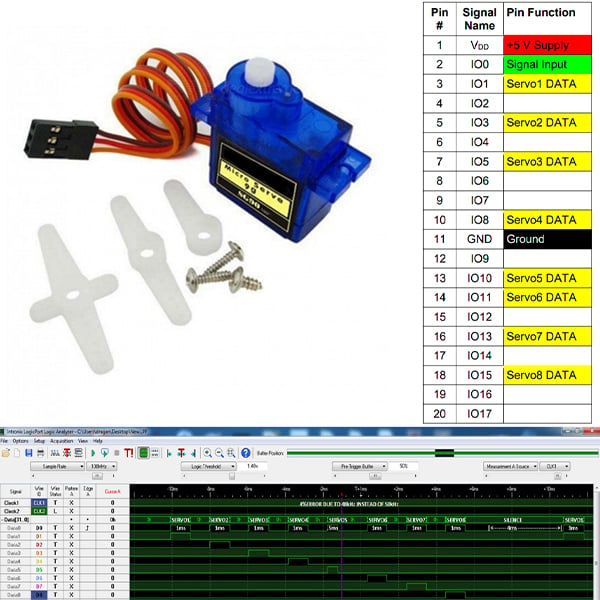Check outhow to create a model servo motor signal demultiplexer using a Dialog GreenPAK IC.

## Hardware

Below we described steps needed to understand how the solution has been programmed to create the servo demultiplexer. However, if you just want to get the result of programming, download GreenPAKâ„¢ Designer software to view the already completed GreenPAK Design file. Plug the GreenPAK Development Kit into your computer and hit the program to design the device.

Servo Motors are widely used for commercial and industrial applications as linear or rotary actuators. In this example the SG90 model is used, which is low cost, consumes little power, and is lightweight. This makes these type of servos ideal for consumer device RC toys such as RF-controlled race cars, airplanes, and others.

However, to properly control a servo motor, the electronic driver must generate appropriate voltage patterns to the servo motor DATA pin. The waveforms should be pulses shorter than 2 milliseconds, repeating every 20 milliseconds for one servo motor.

This project will present the design of one 1 to 8 servo motor signal demultiplexer with the SLG46537V GreenPAK IC. The designed signal demultiplexer would drive up to 8 servo motors on 8 output pins with the multiplexed signal coming in on 1 input pin. The SLG46537V can also integrate additional functionality, such as additional logic or voltage monitoring, depending upon the system requirements.

The following sections will show:

• A servo motor 1 RF channel signal multiplex;
• How to drive 8 servo motors with 1 GreenPAK device.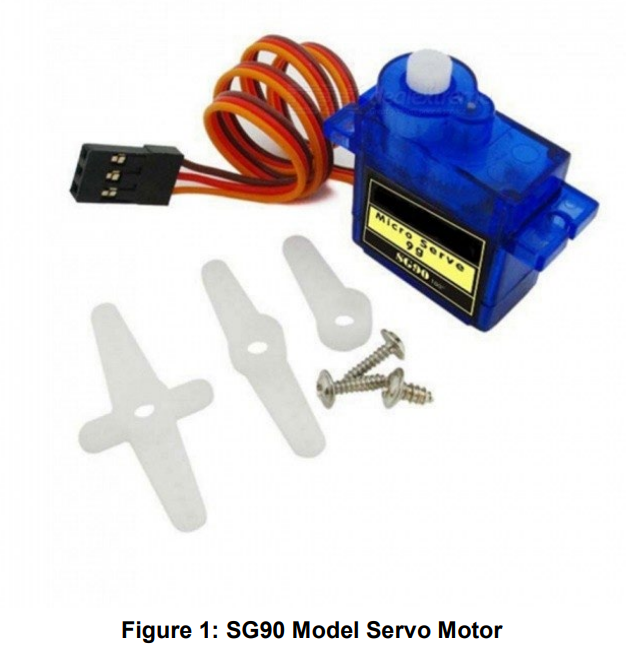## Servo Motor 1 RF Channel Multiplex

The standard for small 9g servo motors is that they operate from 0.5 ms ~ 1.5 ms HIGH pulses with a period of 20 milliseconds. 0.5 ms correlates to 0Â°, 1 ms to 90Â°, and 1.5 ms to 180Â°.

In this way, if we reserve a 2 milliseconds window for each motor pulse, 8 ms x 2 ms can be used for 8 motor pulse windows with the remaining 4 ms silence at the end used for synchronization. Figure 2 shows a time multiplex period of 20 ms for 8 motors, all at 90Â° position (1 ms pulses). Figure 3 shows a time multiplex period of 20 ms for 8 motors, all at 90Â° position (1 ms pulses) except for motor #5 at a 0Â° position (0.5 ms pulse).

Figure 2 and Figure 3 are waveforms of signed digital samples generated by a Python script for a sampling rate of 50,000 Hz.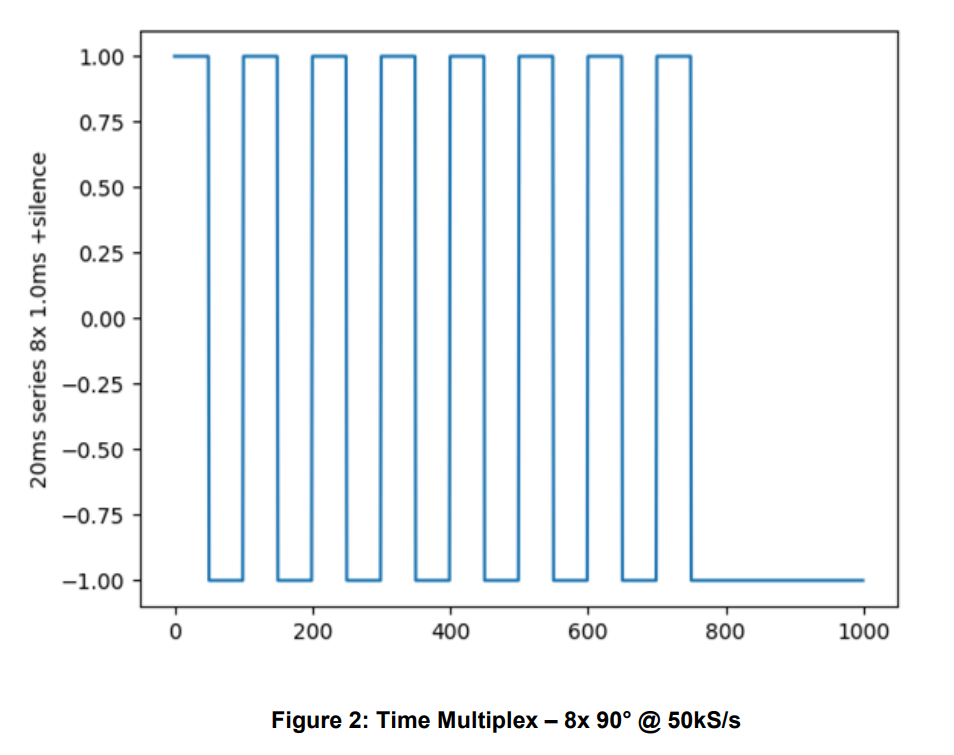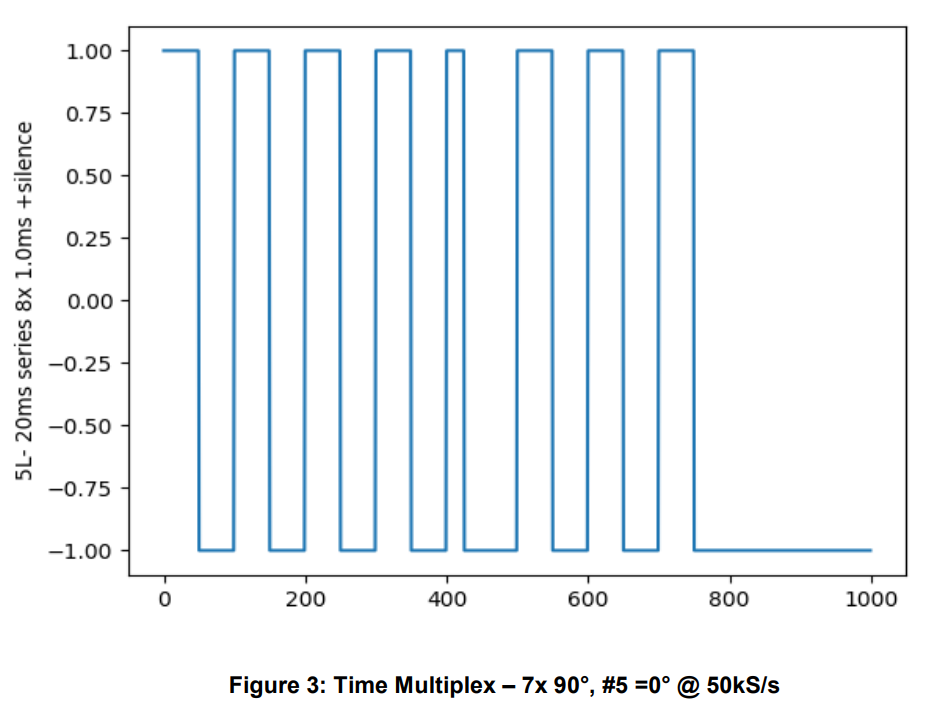## GreenPAK Design Schematic

The schematic of the GreenPAK design is shown in Figure 4. The fundamental blocks of the design are the internal Oscillator, 2x Counter, 2x Filter, 8x Flip-Flop, ASM with reset, 8x Pins with OE and Pull-downs, input pin and supply pins.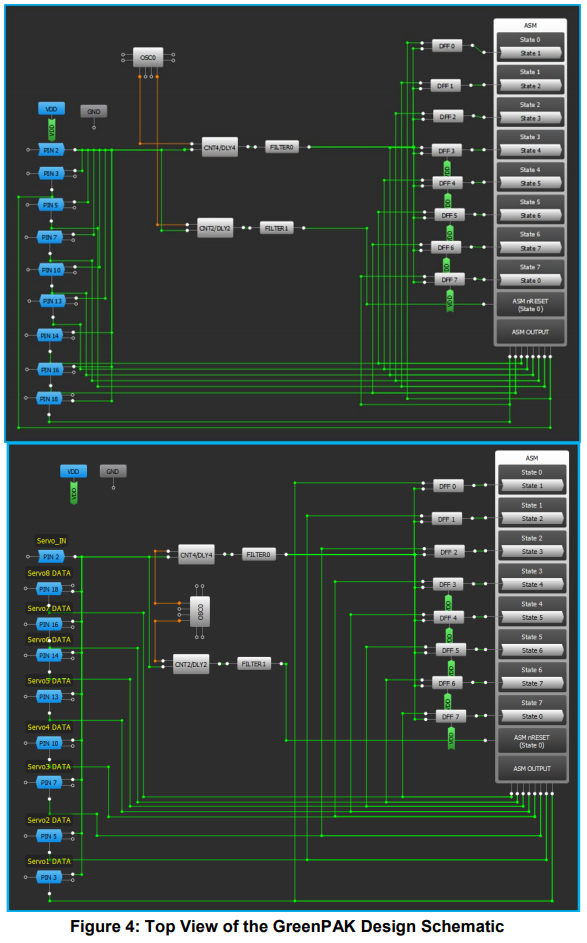GreenPAK Timing Blocks

The OSC0 internal oscillator is used. It drives the CNT2 counter with 2 MHz/8/64 â‰ˆ 3.9 kHz and the CNT4 counter with 2 MHz/8/4 â‰ˆ 62.5 kHz. Settings are shown in Figure 5. CNT2 is set to trigger repeatedly on all falling edges (Delay Mode) and give a Non-inverted OUT after 4.096 ms. This is the 4 ms silence detection at the end of our 20 ms time multiplex period, which resets the ASM to State 0. CNT4 is set to trigger once every falling edge (One Shot Mode) after 96 Î¼s to give a short pulse to all Flip-Flop CLOCK inputs. Only the Flip-Flop of the current state has a HIGH input and will trigger the ASM to transition to the following state.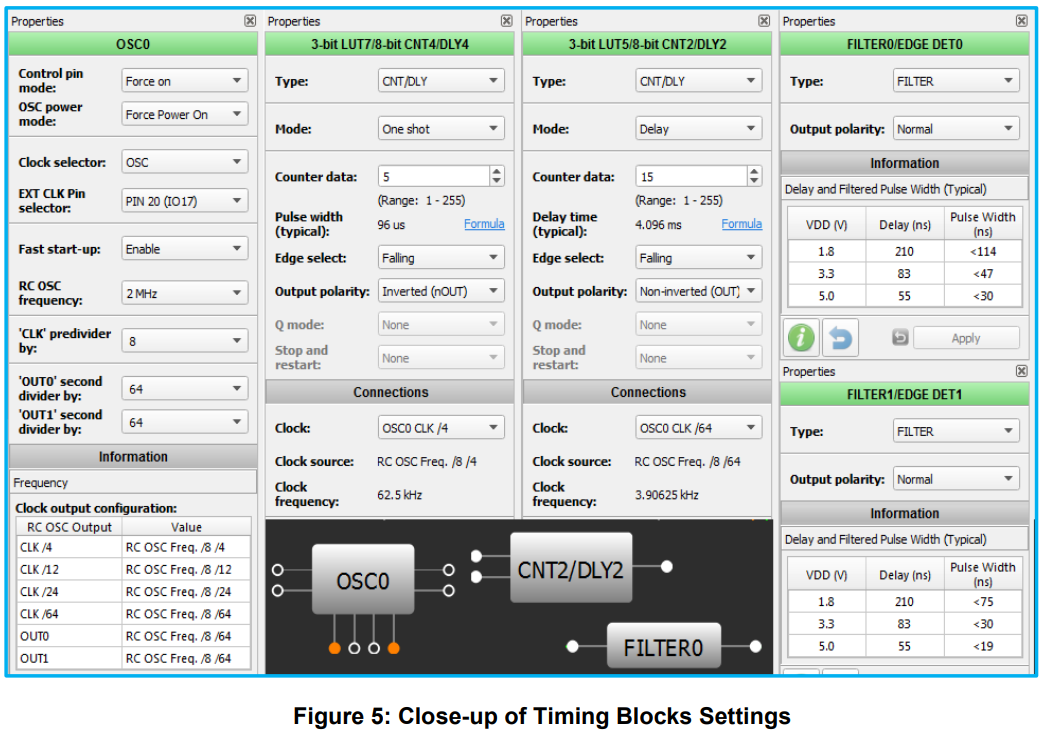GreenPAK ASM

The Flip-Flops in the GreenPAK design are used to change the asynchronous state machine into a synchronous machine. As described in the previous section, CNT/DLY4 delivers a short pulse to all Flip-Flop CLOCK inputs, but only the Flip-Flop of the current state has a HIGH input and will trigger the transition to State +1. ASM runaway thru more than one state is prevented since all the other Flip- Flops were just loaded with LOW inputs, so the ASM has to wait until the next pulse for the next transition. This is necessary since all state transition conditions are the same 1 condition. Settings are shown in Figure 6.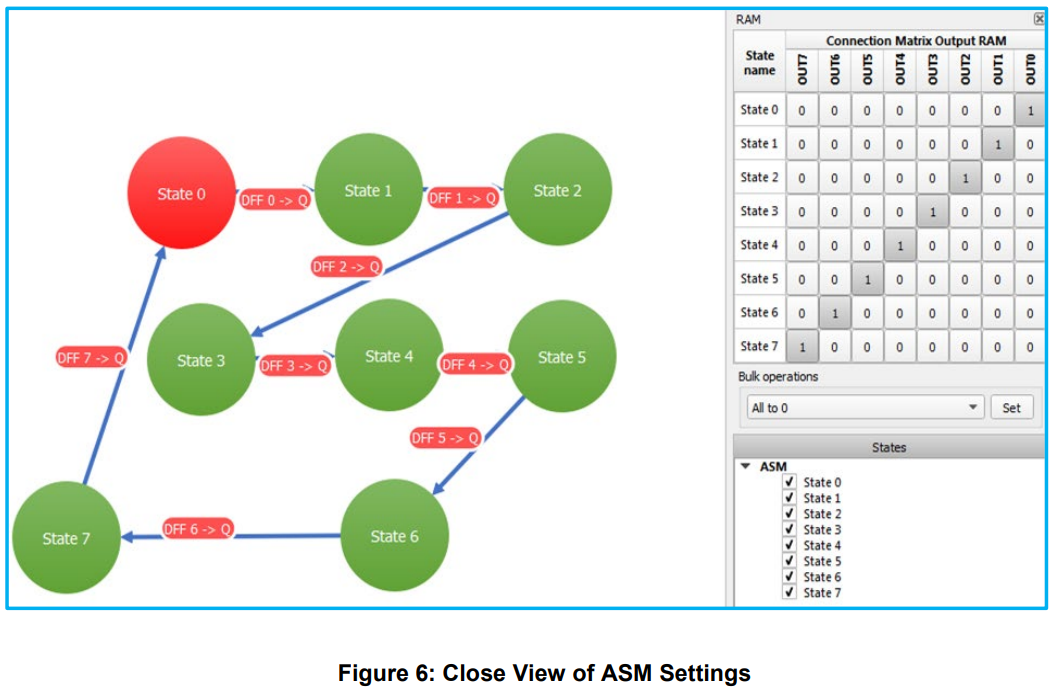## GreenPAK Design Pinout

The Signal input IO0 is configured as a Digital in without Schmitt trigger. The ServoX DATA outputs are configured as 1x Push-Pull at OE = 1, at OE = 0 they are inputs with a 10 k Pull-down resistor. Pinout is shown in Table 1 and Figure 7.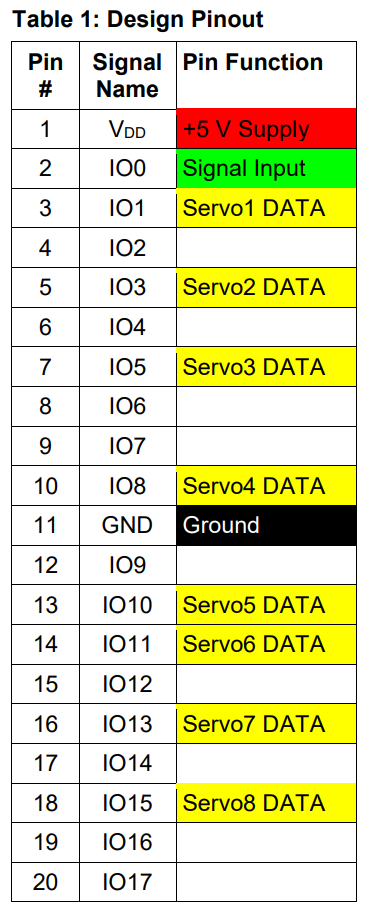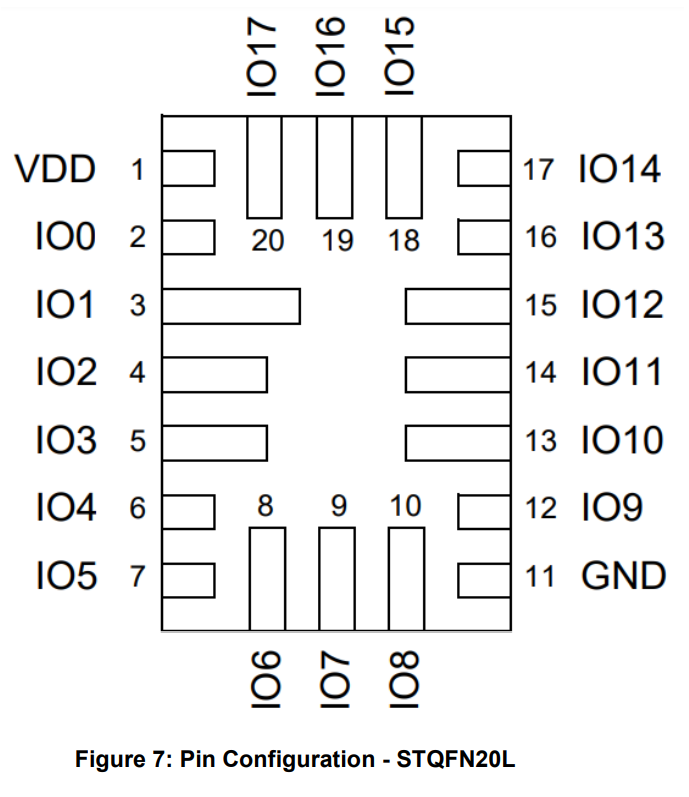## Test Results

The Signal input is driven by an amplified Audio Out signal (0 ~ 5 V) generated by a Python script (in the Appendix below). Figure 8 shows the Signal Input in yellow and the Servo1 DATA output in blue. The generated .wav file has 20 seconds of choreographed servo movements and the GreenPAK design decodes all 8 ServoX DATA signals accordingly which is seen by movements of the servo motors.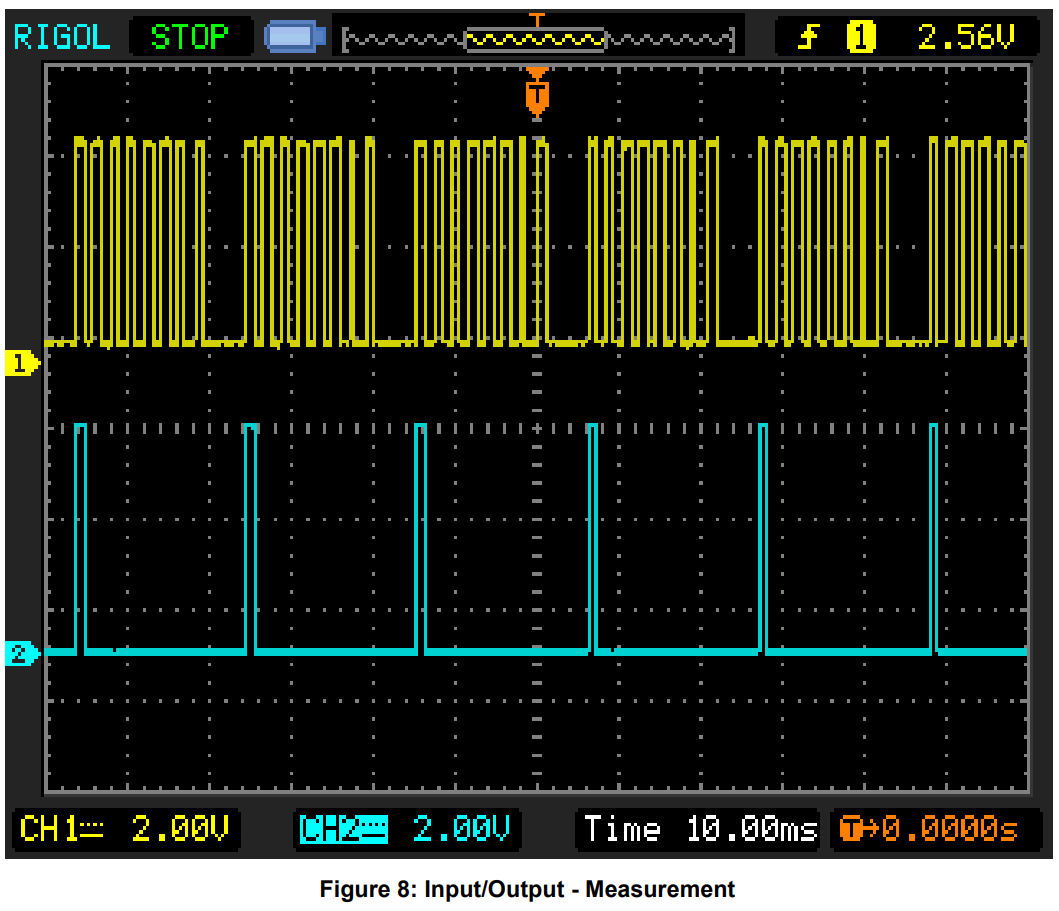## Conclusion and Results Discussion

The Design of a Model Servo Motor Demultiplexer was presented. Through a GreenPAK design with the SLG46537V we have successfully implemented a lightweight, low-power, cost-effective solution. Figure 9 shows the resource usage of the SLG46537V. The design successfully decodes all 8 ServoX DATA signals from one Time Multiplexed signal input - Figure 10.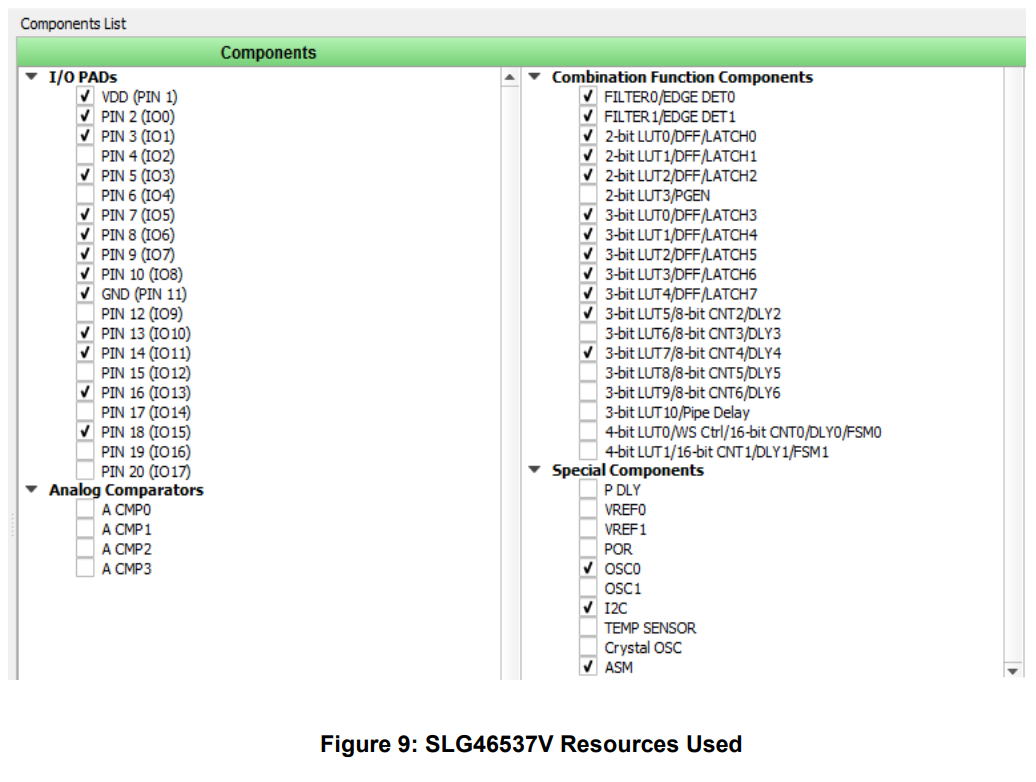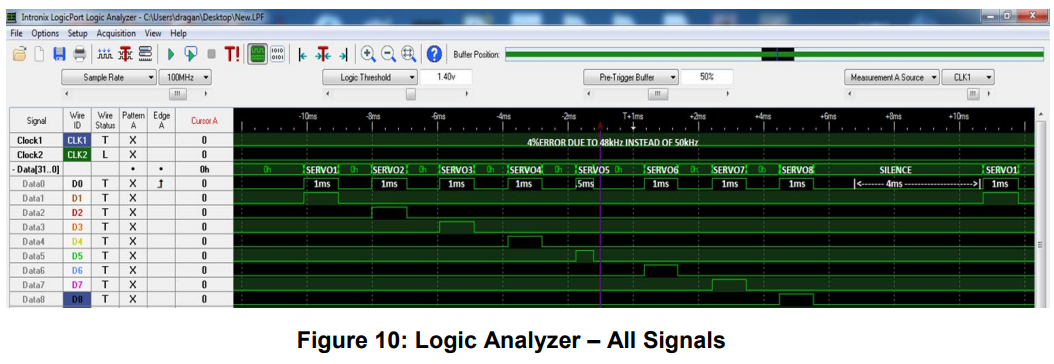## Appendix: Source Code

``````#servoMUX.py###################################################################BEGIN#

import wave

import numpy as np

import matplotlib.pyplot as plt

def repeat48x(series):

return np.concatenate(( series, series, series, series, series, series, series, series, series, series, series, series, series, series, series, series, series, series, series, series, series, series, series, series, series, series, series, series, series, series, series, series, series, series, series, series, series, series, series, series, series, series, series, series, series, series, series, series), axis=None)

silence=-np.ones(200)

pulse10=np.concatenate((np.ones(50), -np.ones(50)), axis=None)

plt.plot(pulse10)

plt.ylabel('pulse 1.0ms')

plt.show()

pulse05=np.concatenate((np.ones(25), -np.ones(75)), axis=None)

plt.plot(pulse05)

plt.ylabel('pulse 0.5ms')

plt.show()

pulse15=np.concatenate((np.ones(75), -np.ones(25)), axis=None)

plt.plot(pulse15)

plt.ylabel('pulse 1.5ms')

plt.show()

#second10

series=np.concatenate((pulse10, pulse10, pulse10, pulse10, pulse10, pulse10, pulse10, pulse10, si- lence), axis=None)

plt.plot(series)

plt.ylabel('20ms series 8x 1.0ms +silence')

plt.show()

second10=repeat48x(series)

plt.plot(second10)

plt.ylabel('1s- 20ms series 8x 1.0ms +silence')

plt.show()

#second1L

series=np.concatenate((pulse05, pulse10, pulse10, pulse10, pulse10, pulse10, pulse10, pulse10, si- lence), axis=None)

plt.plot(series)

plt.ylabel('1L- 20ms series 8x 1.0ms +silence')

plt.show()

second1L=repeat48x(series)

plt.plot(second1L)

plt.ylabel('1L-1s- 20ms series 8x 1.0ms +silence')

plt.show()

#second2L

series=np.concatenate((pulse10, pulse05, pulse10, pulse10, pulse10, pulse10, pulse10, pulse10, si- lence), axis=None)

plt.plot(series)

plt.ylabel('2L- 20ms series 8x 1.0ms +silence')

plt.show()

second2L=repeat48x(series)

plt.plot(second2L)

plt.ylabel('2L-1s- 20ms series 8x 1.0ms +silence')

plt.show()

#second3L

series=np.concatenate((pulse10, pulse10, pulse05, pulse10, pulse10, pulse10, pulse10, pulse10, si- lence), axis=None)

plt.plot(series)

plt.ylabel('3L- 20ms series 8x 1.0ms +silence')

plt.show()

second3L=repeat48x(series)

plt.plot(second3L)

plt.ylabel('3L-1s- 20ms series 8x 1.0ms +silence')

plt.show()

#second4L

series=np.concatenate((pulse10, pulse10, pulse10, pulse05, pulse10, pulse10, pulse10, pulse10, si- lence), axis=None)

plt.plot(series)

plt.ylabel('4L- 20ms series 8x 1.0ms +silence')

plt.show()

second4L=repeat48x(series)

plt.plot(second4L)

plt.ylabel('4L-1s- 20ms series 8x 1.0ms +silence')

plt.show()

#second5L

series=np.concatenate((pulse10, pulse10, pulse10, pulse10, pulse05, pulse10, pulse10, pulse10, si- lence), axis=None)

plt.plot(series)

plt.ylabel('5L- 20ms series 8x 1.0ms +silence')

plt.show()

second5L=repeat48x(series)

plt.plot(second5L)

plt.ylabel('5L-1s- 20ms series 8x 1.0ms +silence')

plt.show()

#second6L

series=np.concatenate((pulse10, pulse10, pulse10, pulse10, pulse10, pulse05, pulse10, pulse10, si- lence), axis=None)

plt.plot(series)

plt.ylabel('6L- 20ms series 8x 1.0ms +silence')

plt.show()

second6L=repeat48x(series)

plt.plot(second6L)

plt.ylabel('6L-1s- 20ms series 8x 1.0ms +silence')

plt.show()

#second7L

series=np.concatenate((pulse10, pulse10, pulse10, pulse10, pulse10, pulse10, pulse05, pulse10, silence), axis=None)

plt.plot(series)

plt.ylabel('7L- 20ms series 8x 1.0ms +silence')

plt.show()

second7L=repeat48x(series)

plt.plot(second7L)

plt.ylabel('7L-1s- 20ms series 8x 1.0ms +silence')

plt.show()

#second8L

series=np.concatenate((pulse10, pulse10, pulse10, pulse10, pulse10, pulse10, pulse10, pulse05, silence), axis=None)

plt.plot(series)

plt.ylabel('8L- 20ms series 8x 1.0ms +silence')

plt.show()

second8L=repeat48x(series)

plt.plot(second8L)

plt.ylabel('8L-1s- 20ms series 8x 1.0ms +silence')

plt.show()

w=wave.open("servo.wav", 'wb')
w.setnchannels(1)
w.setsampwidth(1)
w.setframerate(48000)
#w.setnframes(24000)
#w.setcomptype('NONE', 'wav')
w.writeframesraw(100*second10.astype(np.int8))
w.writeframesraw(100*second10.astype(np.int8))
w.writeframesraw(100*second10.astype(np.int8))
w.writeframesraw(100*second10.astype(np.int8))
w.writeframesraw(100*second1L.astype(np.int8))
w.writeframesraw(100*second2L.astype(np.int8))
w.writeframesraw(100*second3L.astype(np.int8))
w.writeframesraw(100*second4L.astype(np.int8))
w.writeframesraw(100*second5L.astype(np.int8))
w.writeframesraw(100*second6L.astype(np.int8))
w.writeframesraw(100*second7L.astype(np.int8))
w.writeframesraw(100*second8L.astype(np.int8))
w.writeframesraw(100*second10.astype(np.int8))
w.writeframesraw(100*second10.astype(np.int8))
w.writeframesraw(100*second10.astype(np.int8))
w.writeframesraw(100*second10.astype(np.int8))
w.close()

#servoMUX.py#####################################################################END#``````